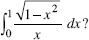# AP Calculus BC Practice Test 19

### Test Information9 questions27 minutes

Calculator Allowed

1. When partial fractions are used, the decomposition of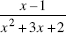is equal to

2.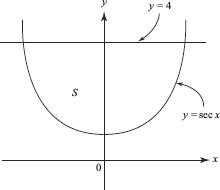The region S in the figure above is bounded by y = sec x and y = 4. What is the volume of the solid formed when S is rotated about the x-axis?

3. The series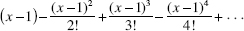converges

4. If f (x) is continuous at the point where x = a, which of the following statements may be false?

5. A Maclaurin polynomial is to be used to approximate y = sin x on the interval - π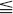xπ. What is the least number of terms needed to guarantee no error greater than 0.1?

6. Find the area bounded by the y-axis and the curve defined parametrically by x(t) = 4 - t2, y(t) = 2t.

7. Which series diverges?

8. If x = 2t - 1 and y = 3 - 4t2, then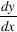is

9. For the substitution x = sin θ, which integral is equivalent to